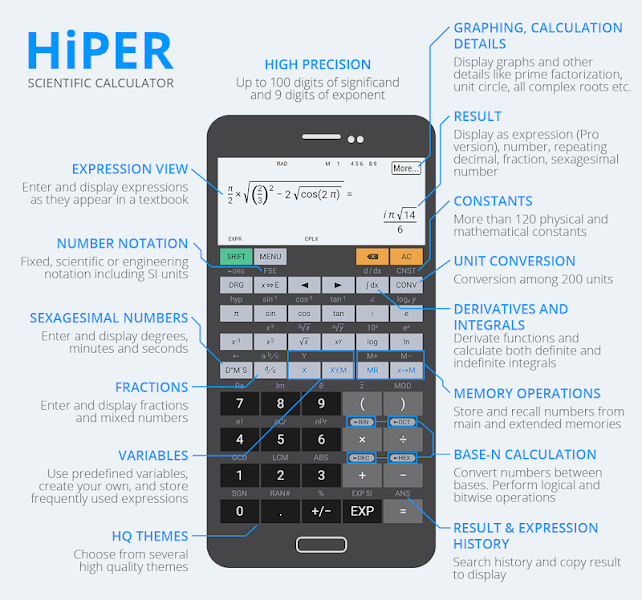# HiPER Calc Pro APK 8.1.2 (MOD, Patched)App Name HiPER Calc Pro HiPER Development Studio Tools 3.1MB 8.1.2 build 142 Paid/Patched 4.1 and upDecember 28, 2020 (3 weeks ago)
• Substitution method for equation systems
• Numerical method for equations
• ANS key renamed to HISTORY
• New detail: derivatives of a result
• New detail: indefinite integrals of a result
• Graph of an equation with two unknowns
• Graph of equation system with two unknowns
• New special values in graph: minima, maxima and inflex points
• Warnings in ambiguous situations (e.g. probably wrong angular unit)
• Paid/Patched

HiPER Calc Pro APK is an advanced version of the HiPER Scientific Calculator.

The calculator has up to 100 digits of significand and 9 digits of exponent. It detects repeating decimals and numbers can be also entered as fractions or converted to fractions.

You can write expressions in a natural way and watch your calculations. The result is displayed as a number, simplified expression, etc.

The calculator has several layouts suitable for various screen sizes:
– “pocket” for small devices
– “compact” for smartphones (in portrait and landscape orientation)
– “expanded” for tablets

A multiline display can be turned on in tablets to show the complete history of calculations and to provide access to the previous results.

Users can choose from several high-quality themes.## HiPER Calc Pro APK has several functions, such as:

• basic arithmetic operations including percentage, modulo, and negation;
• fractions (in the expression mode any expression including nested fractions can be entered as a numerator and a denominator);
• mixed numbers;
• periodic numbers and their conversion to fractions;
• unlimited number of braces;
• operator priority;
• repeated operations;
• equations
• variables and symbolic computation;
• derivatives and integrals;
• graphs of functions and integral area, 3D graphs;
• calculation details – extended information about a calculation like all complex roots, unit circle, etc.;
• complex numbers
• conversion between rectangular and polar coordinates
• advanced number operations such as random numbers, combinations, permutations, common greatest divisor, etc.;
• trigonometric and hyperbolic functions;
• powers, roots, logarithms, etc.;
• degrees, minutes, and seconds conversion;
• fixed point, scientific and engineering display format;
• display exponent as SI units prefix;
• memory operations with 10 extended memories;
• clipboard operations with various clipboard formats;
• result in history;
• binary, octal, and hexadecimal numeral systems;
• logical operations;
• bitwise shifts and rotations;
• haptic feedback;
• more than 90 physical constants;
• conversion among 200 units;
• Reverse Polish notation.

The calculator has many settings to manage the full-screen mode, decimal and thousand separators, etc.

All features are described with built-in help.